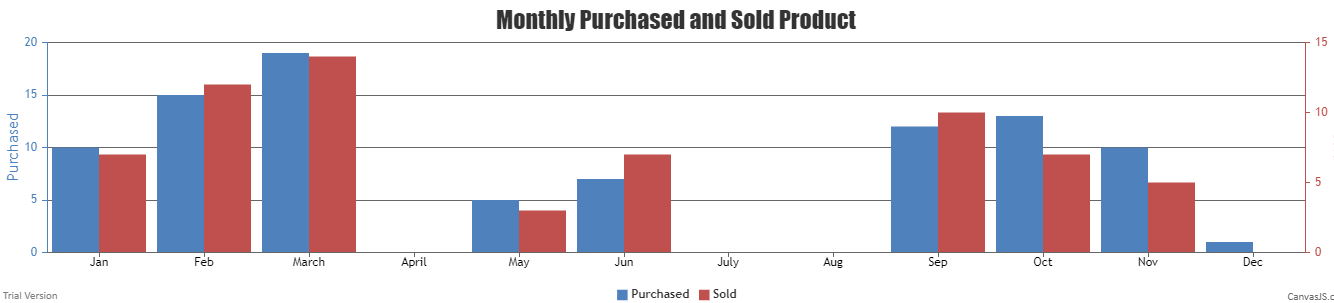# How to make dynamic chart in PHP using canvasJS?

• Last Updated : 24 May, 2019

CanvasJS is a JavaScript library which is used to make a different type of charts easily for the webpage. For example Bar chart, Pie chart, Column chart, Area chart, Line chart, etc.

Let’s take an example that needs to create a graph in which we can display monthly sold and purchased products. We will consider two arrays which we can also consider from our database. Once we get the data from the database and stored in arrays, it can easily draw a dynamic graph using library canvasJS.

Create a file and save it in a project folder. The filename chart_sample.php contains data in the form of an array, where the first array represents the purchased products and the second array represents the sols products list. Now, use canvasJS to draw a graph.

Example:

 ` ``"Jan"``, ``"y"``=> ``\$purchased``),``    ``array``(``"label"``=> ``"Feb"``, ``"y"``=> ``\$purchased``),``    ``array``(``"label"``=> ``"March"``, ``"y"``=> ``\$purchased``),``    ``array``(``"label"``=> ``"April"``, ``"y"``=> ``\$purchased``),``    ``array``(``"label"``=> ``"May"``, ``"y"``=> ``\$purchased``),``    ``array``(``"label"``=> ``"Jun"``, ``"y"``=> ``\$purchased``),``    ``array``(``"label"``=> ``"July"``, ``"y"``=> ``\$purchased``),``    ``array``(``"label"``=> ``"Aug"``, ``"y"``=> ``\$purchased``),``    ``array``(``"label"``=> ``"Sep"``, ``"y"``=> ``\$purchased``),``    ``array``(``"label"``=> ``"Oct"``, ``"y"``=> ``\$purchased``),``    ``array``(``"label"``=> ``"Nov"``, ``"y"``=> ``\$purchased``),``    ``array``(``"label"``=> ``"Dec"``, ``"y"``=> ``\$purchased``)``);``  ` `// Data to draw graph for sold products``\$dataPoints2` `= ``array``(``    ``array``(``"label"``=> ``"Jan"``, ``"y"``=> ``\$sold``),``    ``array``(``"label"``=> ``"Feb"``, ``"y"``=> ``\$sold``),``    ``array``(``"label"``=> ``"March"``, ``"y"``=> ``\$sold``),``    ``array``(``"label"``=> ``"April"``, ``"y"``=> ``\$sold``),``    ``array``(``"label"``=> ``"May"``, ``"y"``=> ``\$sold``),``    ``array``(``"label"``=> ``"Jun"``, ``"y"``=> ``\$sold``),``    ``array``(``"label"``=> ``"July"``, ``"y"``=> ``\$sold``),``    ``array``(``"label"``=> ``"Aug"``, ``"y"``=> ``\$sold``),``    ``array``(``"label"``=> ``"Sep"``, ``"y"``=> ``\$sold``),``    ``array``(``"label"``=> ``"Oct"``, ``"y"``=> ``\$sold``),``    ``array``(``"label"``=> ``"Nov"``, ``"y"``=> ``\$sold``),``    ``array``(``"label"``=> ``"Dec"``, ``"y"``=> ``\$sold``)``);``      ` `?>``  ` `````  ``    ````    `````` ` ```    ``
`````

Output:Reference: CanvasJS

My Personal Notes arrow_drop_up